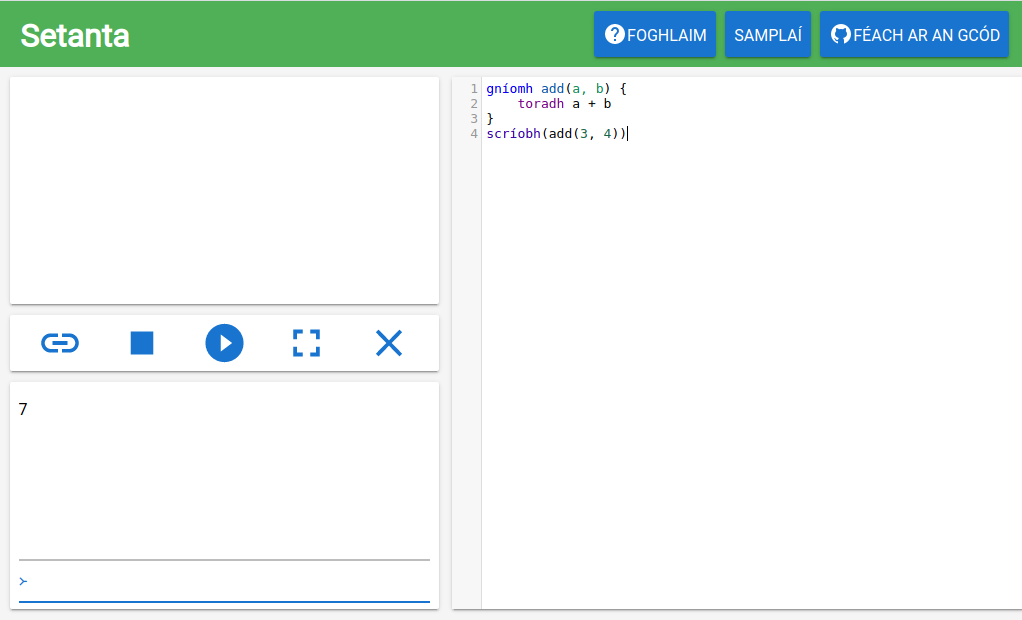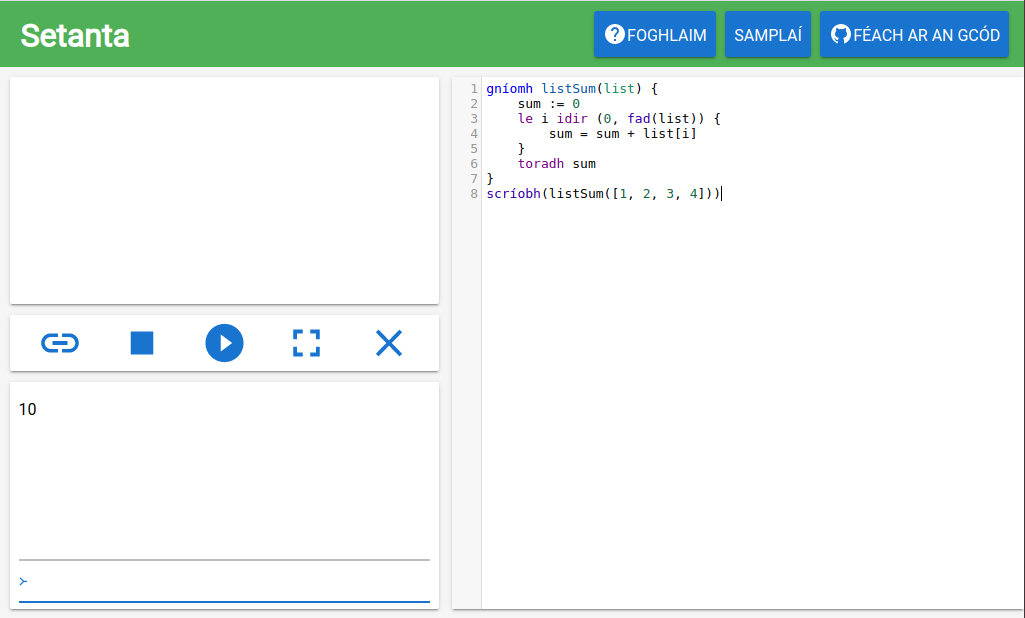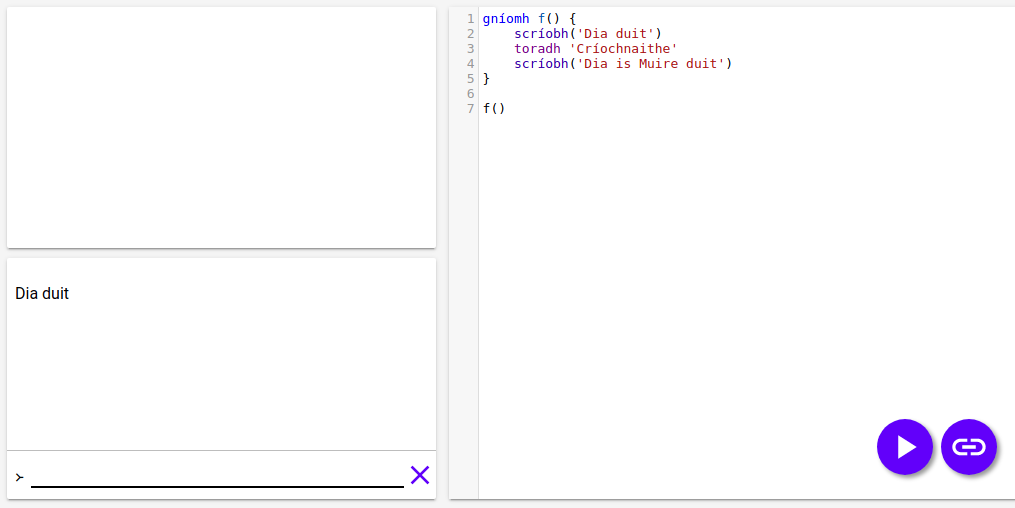# Results

## Actions with results

To explain results, we will look at some actions that come with Setanta. For example, Setanta comes with the action `fad`. You use `fad` to get the length of a list or text. “fad” translates as length in English.

``````liosta := [0, 1, 2]

ainm := 'Cú Chulainn'

When you use `fad` you get a result back, specifically the length of the list or the length of the text.

In the same way, the action `go_uimh` has a result. You use `go_uimh` to convert text to a number. For example:

``go_uimh('123') == 123``

`123` is the result of the `go_uimh` action.

## Our own functions.

It’s clear that results are very useful. We can create actions with results with the word `toradh` (meaning “result”). Say we want to create an action to add two numbers. We can write this:

``````gníomh add(a, b) {
}``````

Now when we call `add` with `add(a, b)`, we get back `a + b`. Try it out now!## A more complicated example

Now we mix loops, actions and results to create an action to add every number in a list.

At first we create the outline of the action.

``````gníomh listSum(list) {
}``````

Now we want to go over every number in the variable `list`. We have to use a list to go from the start of the list to the end. We first look at `list`, then `list`, `list` etc. until the end. We use `fad` to get the length of the list

``````gníomh listSum(list) {
le i idir (0, fad(list)) {

}
}``````

Now we create a variable to store the sum.

``````gníomh listSum(list) {
sum := 0
le i idir (0, fad(list)) {

}
}``````

Now, in the loop, when we are looking at the the number of the list in position `i`, we add `list[i]` and the variable `sum` together and we put the result back in `sum`.

``````gníomh listSum(list) {
sum := 0
le i idir (0, fad(list)) {
sum = sum + list[i]
}
}``````

When the loop is finished the value of the variable `sum` is the sum of the whole list. Now there’s nothing left to do but to put this value in the result of the action with the word `toradh`.

``````gníomh listSum(list) {
sum := 0
le i idir (0, fad(list)) {
sum = sum + list[i]
}
}``````

We can run this code and see that it’s working! Try `listSum([1, 2, 3, 4])` and see that it returns 10, which is 1 + 2 + 3 + 4.## NB

The word `toradh` works like the word `bris`. When Setanta follows `toradh`, the action stops completely. To explain this, look at this action:

``````gníomh f() {
scríobh('Dia duit')The program didn’t write ‘Dia is Muire duit’, because it stopped when it reached the line `toradh 'Críochnaithe'`.